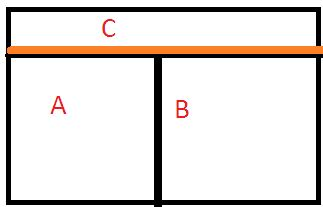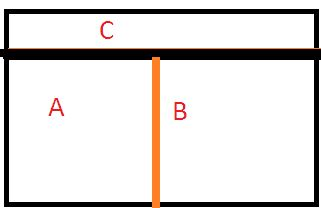# PhysicsTeacher.in

## High School Physics BLOG - online class notesRSS Feed

# Zeroth Law of Thermodynamics and Concepts of Thermal Equilibrium

We will begin with a briefing on the definition of Thermodynamics and the concepts of Thermal Equilibrium in the context of Thermodynamics. Thermodynamics is the branch of physics that deals with the concepts of heat and temperature and the inter-conversion of heat and the other forms of energy. And Equilibrium in Thermodynamics is availed when the variables of the systems don’t change with time. For example, say a gas inside a closed rigid container is insulated from its surroundings. And as a result, say, its pressure, volume, temperature, mass and composition remain unchanged. In this case, we can say that the gas has attained a state of equilibrium. Zeroth Law of Thermodynamics throws light on a major condition of thermal equilibrium among systems.

## Zeroth law of Thermodynamics

This states that if two systems are in thermal equilibrium with a third system separately then they (the first 2 systems) are in thermal equilibrium with each other as well. Let’s discuss in details. We will a 2 step process to understand the basic concepts.

#### Step 1: Thermal Equilibrium in ThermodynamicsStep 1

Let’s take 3 systems, A, B and C.

System A and B are isolated by an adiabatic wall (no conduction of heat), but each of these A and B is individually isolated to a 3rd system C by a conducting wall.

As time passes, after some time A will come to thermal equilibrium with C and similarly system B will have the same equilibrium state with C separately.

Now, understanding the fact that thermal equilibrium means temperature equality, we can write the following equations, as per the above law stated:

If Ta = Tc …………….. (1) and
Tb = Tc …………………….(2)
[ Ta, Tb and Tc are the temperature of the systems A,B and C respectively.]

#### Step 2: Thermal Equilibrium in Thermodynamicsstep 2

Now if we interchange the nature of the 2 walls, i.e. between A and B now the wall would be conducting and the other wall between C and other 2 systems become adiabatic, then what is going to happen?

The wall separating C with others being adiabatic, there is no possible interaction with C. but there is ample scope of heat transfer now between A and B.

But, it is found that there is no further change of states for A and B. That means they are now in Thermal Equilibrium with each other.

That means as per the Zeroth Law of Thermodynamics, we can say, Ta = Tb .

### zeroth law of Thermodynamics: Summing up

So summing up the above 2 steps we can write,

If Ta = Tc …………….. (1) and
Tb = Tc …………………….(2)

then Ta = Tb …………(3) [ at thermal equilibrium]……showcasing the zeroth law of Thermodynamics which states if two systems are in thermal equilibrium with a third system separately then the first 2 systems are in thermal equilibrium with each other as well.

###### Interesting Related Post:

First Law of Thermodynamics

Thermal Conductivity – definition, dimension, concepts, values

error: Content is protected !!